Equations reduced to pair of linear equations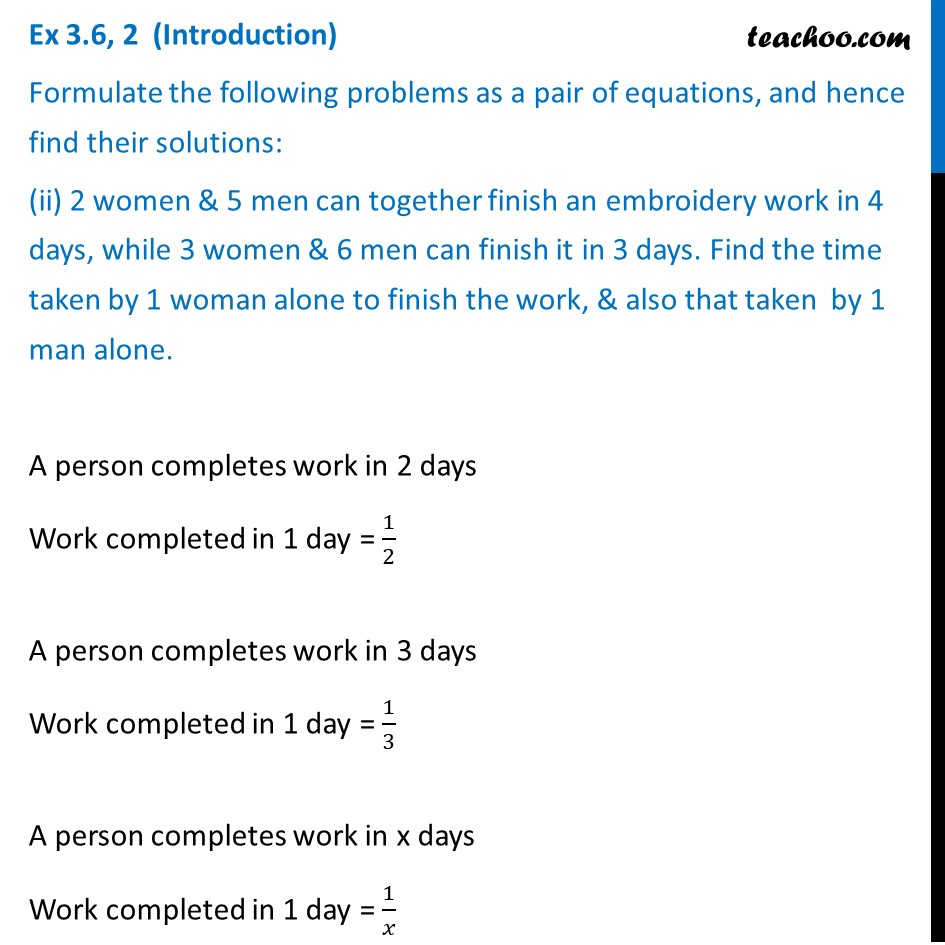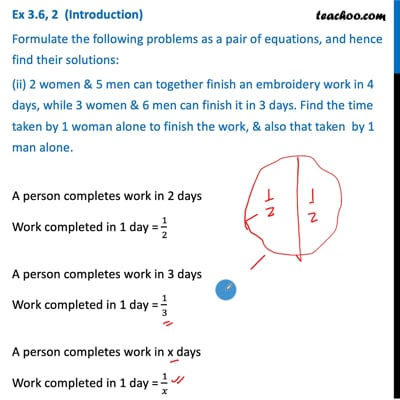This video is only available for Teachoo black users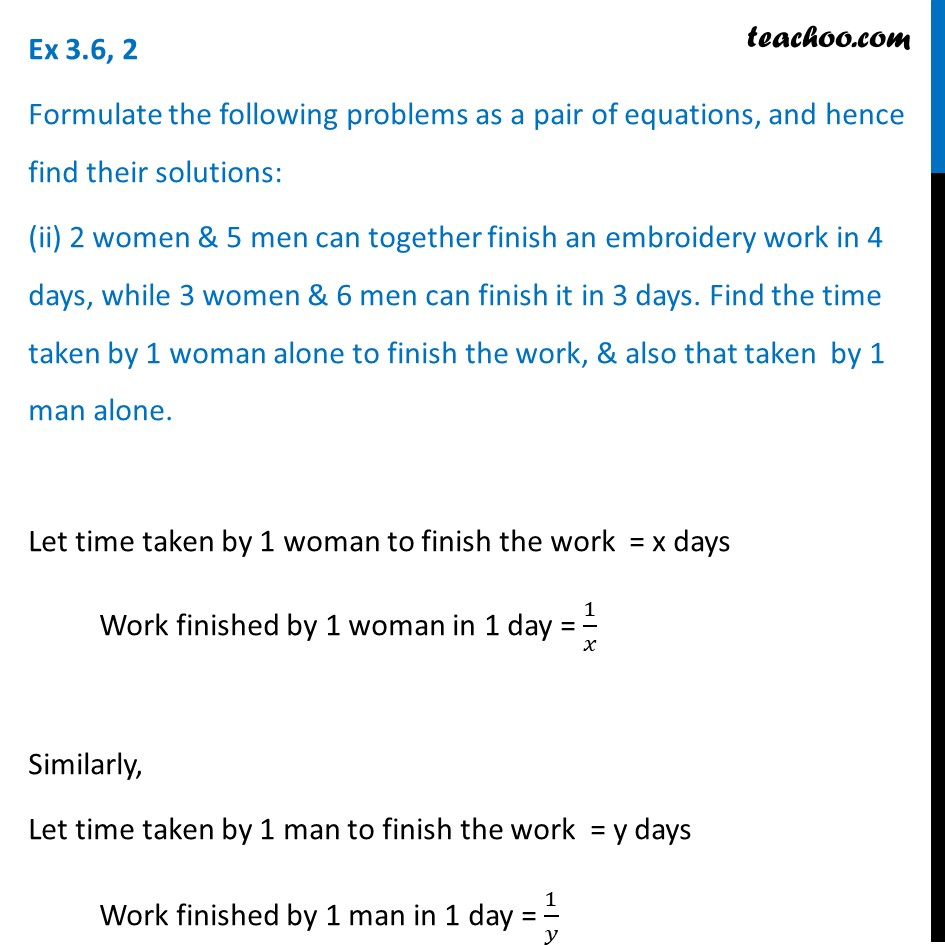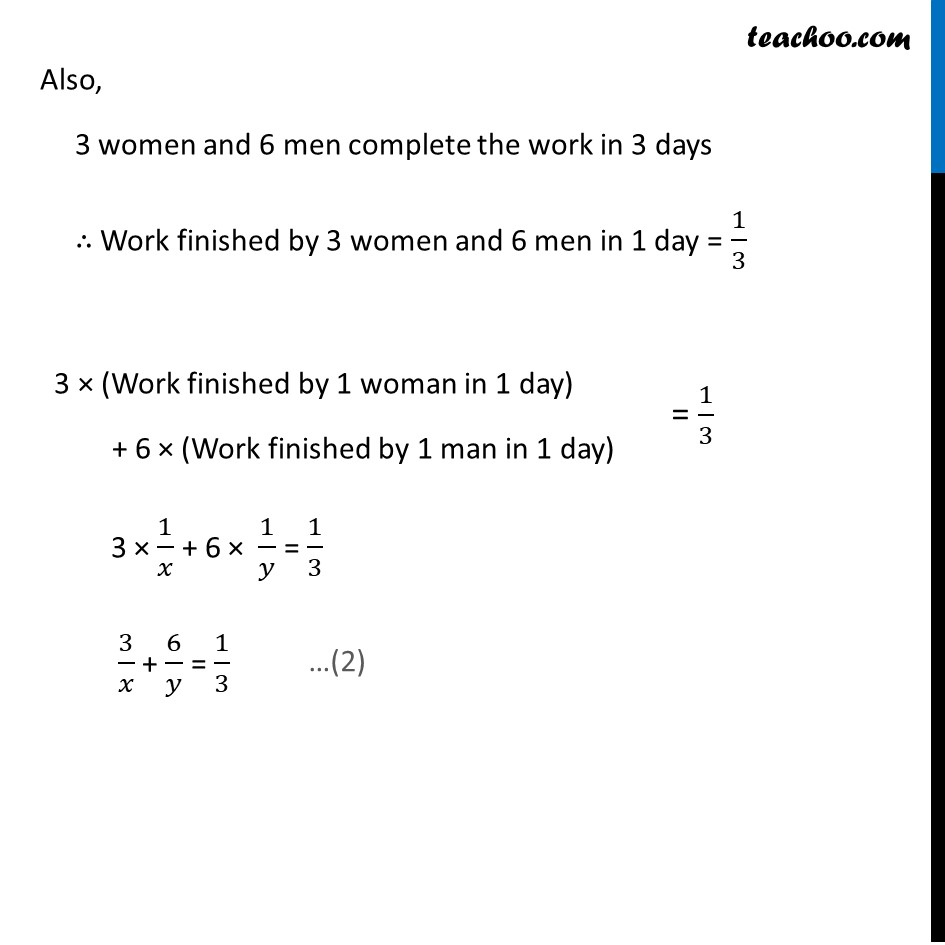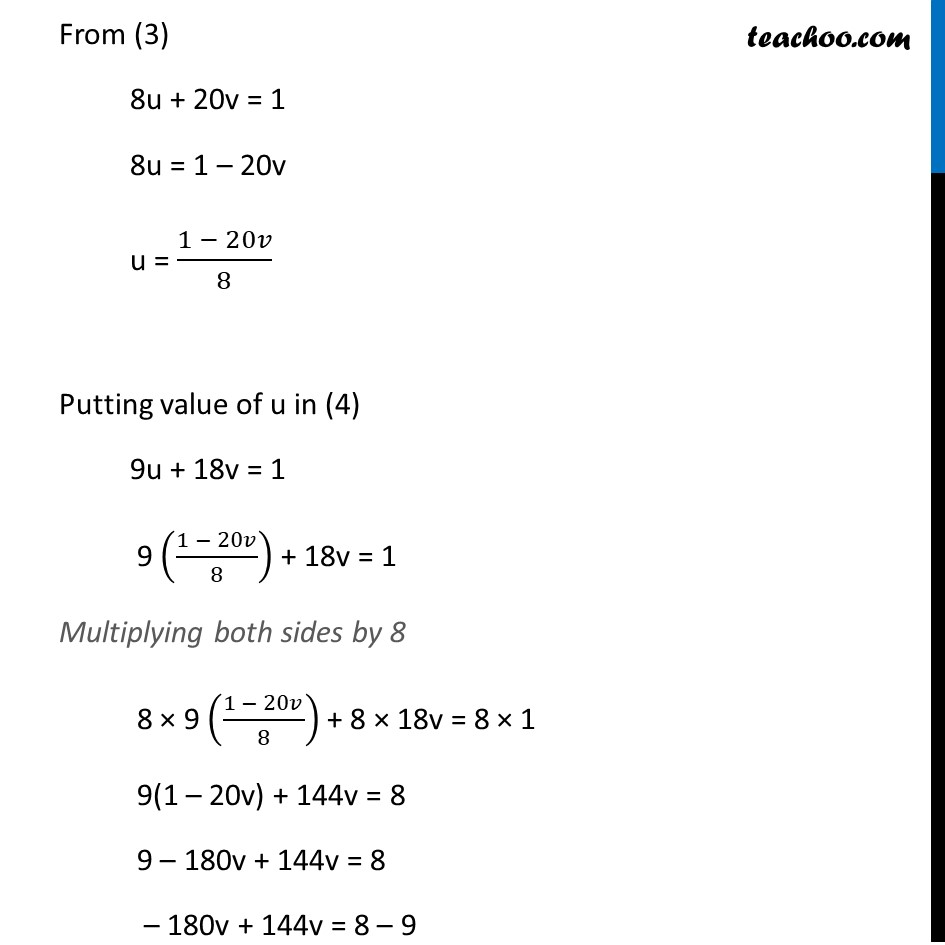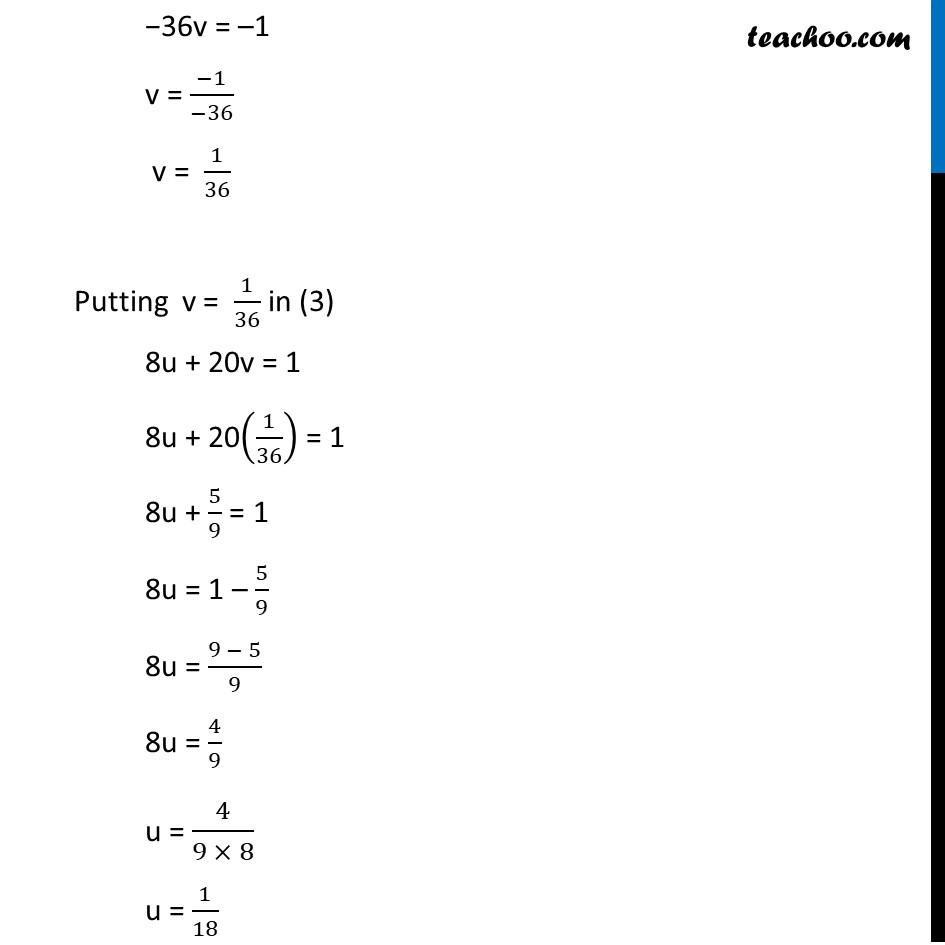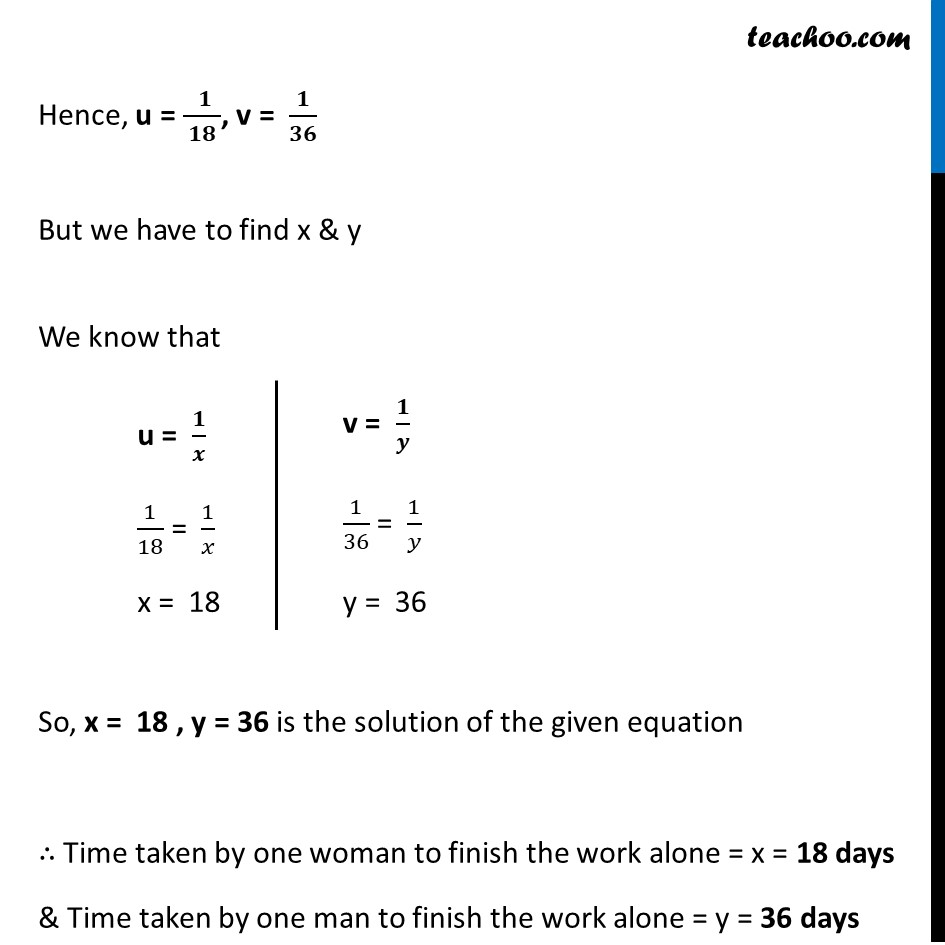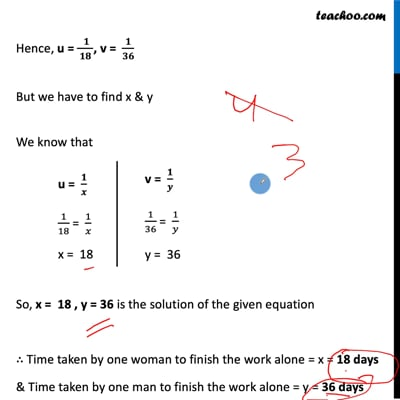This video is only available for Teachoo black users

Learn in your speed, with individual attention - Teachoo Maths 1-on-1 Class

### Transcript

Question 2 (Introduction) Formulate the following problems as a pair of equations, and hence find their solutions: (ii) 2 women & 5 men can together finish an embroidery work in 4 days, while 3 women & 6 men can finish it in 3 days. Find the time taken by 1 woman alone to finish the work, & also that taken by 1 man alone. A person completes work in 2 days Work completed in 1 day = 1/2 A person completes work in 3 days Work completed in 1 day = 1/3 A person completes work in x days Work completed in 1 day = 1/𝑥 We will use this theory in our question Question 2 Formulate the following problems as a pair of equations, and hence find their solutions: (ii) 2 women & 5 men can together finish an embroidery work in 4 days, while 3 women & 6 men can finish it in 3 days. Find the time taken by 1 woman alone to finish the work, & also that taken by 1 man alone. Let time taken by 1 woman to finish the work = x days Work finished by 1 woman in 1 day = 1/𝑥 Similarly, Let time taken by 1 man to finish the work = y days Work finished by 1 man in 1 day = 1/𝑦 Given that 2 women and 5 men complete the work in 4 days ∴ Work finished by 2 women and 5 men in 1 day = 1/4 2 × (Work finished by 1 woman in 1 day) + 5 × (Work finished by 1 man in 1 day) 2 × 1/𝑥 + 5 × 1/𝑦 = 1/4 2/𝑥 + 5/𝑦 = 1/4 Also, 3 women and 6 men complete the work in 3 days ∴ Work finished by 3 women and 6 men in 1 day = 1/3 3 × (Work finished by 1 woman in 1 day) + 6 × (Work finished by 1 man in 1 day) 3 × 1/𝑥 + 6 × 1/𝑦 = 1/3 3/𝑥 + 6/𝑦 = 1/3 Our two equations become 2/𝑥 + 5/𝑦 = 1/4 …(1) 3/𝑥 + 6/𝑦 = 1/3 …(2) So, our equations become 2u + 5v = 1/4 4(2u + 5v) = 1 8u + 20v = 1 3u + 6v = 1/3 3(3u + 6v) = 1 9u + 18v = 1 Hence, our equations are 8u + 20v = 1 …(3) 9u + 18v = 1 …(4) From (3) 8u + 20v = 1 8u = 1 – 20v u = (1 − 20𝑣)/8 Putting value of u in (4) 9u + 18v = 1 9 ((1 − 20𝑣)/8) + 18v = 1 Multiplying both sides by 8 8 × 9 ((1 − 20𝑣)/8) + 8 × 18v = 8 × 1 9(1 – 20v) + 144v = 8 9 – 180v + 144v = 8 – 180v + 144v = 8 – 9 −36v = –1 v = (−1)/(−36) v = 1/36 Putting v = 1/36 in (3) 8u + 20v = 1 8u + 20(1/36) = 1 8u + 5/9 = 1 8u = 1 – 5/9 8u = (9 − 5)/9 8u = 4/9 u = 4/(9 × 8) u = 1/18 Hence, u = ( 𝟏)/( 𝟏𝟖 ), v = 𝟏/𝟑𝟔 But we have to find x & y We know that u = 𝟏/𝒙 1/18 = 1/𝑥 x = 18 v = 𝟏/𝒚 1/36 = 1/𝑦 y = 36 So, x = 18 , y = 36 is the solution of the given equation ∴ Time taken by one woman to finish the work alone = x = 18 days & Time taken by one man to finish the work alone = y = 36 days